# Hexagon shape

Here you will learn about the hexagon shape including its properties, the different types of hexagon shapes and how to solve problems involving hexagon shapes.

Students will first learn about the hexagon shape as part of geometry in elementary school.

## What is the hexagon shape?

A hexagon shape is a closed two-dimensional polygon made up of six straight sides.

Hexagons have six vertices (corners), six interior angles and six exterior angles. The name hexagon is divided into ‘hex’ meaning six, and ‘gonia’ meaning apex or corners.

Hexagon shapes can have the same or different dimensions of length. A regular hexagon shape has six sides that are equal in length, six interior angles that are equal in size and six exterior angles that are equal in size.

Some examples of real life regular hexagons include floor tiles and honeycomb in beehives which form tessellating hexagonal patterns.Snowflakes are also hexagonal patterns.

An irregular hexagon shape has six sides, six interior angles and six exterior angles that are not all equal.

Hexagons can also be convex or concave.

Convex hexagonsConcave hexagons

A convex hexagon shape has interior
angles that all measure less than 180^{\circ}.

Convex hexagons can be regular or
irregular. All the vertices (corners) of a
convex hexagon are pointed outwards.

For example,

A concave hexagon shape has at least
one interior angle that is greater than
180^{\circ}.

Concave hexagons have at least one
vertex that points inwards.

For example,

### Sides of a hexagon shape

All hexagon shapes have six straight edges that form a closed shape.

Regular hexagons have six equal sides.

Irregular hexagons have six sides that are not all equal in length or six interior angles that are not equal in size, or both.

By adding the lengths of all six sides of a hexagon, you get the perimeter of the hexagon.

In a regular hexagon, if you know the value of the perimeter, you can calculate the length of each side by dividing the perimeter by six.

For example,

The regular hexagon shape below has a perimeter of 108 \, cm.

You can calculate the length of each side by,

108 \div 6=18.

So, the length of each side of the regular hexagon shape is 18 \, cm.

### Angles of a hexagon shape

All polygons have interior (inside) and exterior (outside) angles. A pair of interior and exterior angles form a straight line and therefore add to 180^{\circ}.

Interior angles are the angles inside a polygon formed at a vertex where two sides meet.

Exterior angles are the angles between a polygon and the extended line from the vertex (corner) of the polygon.

### What is the hexagon shape?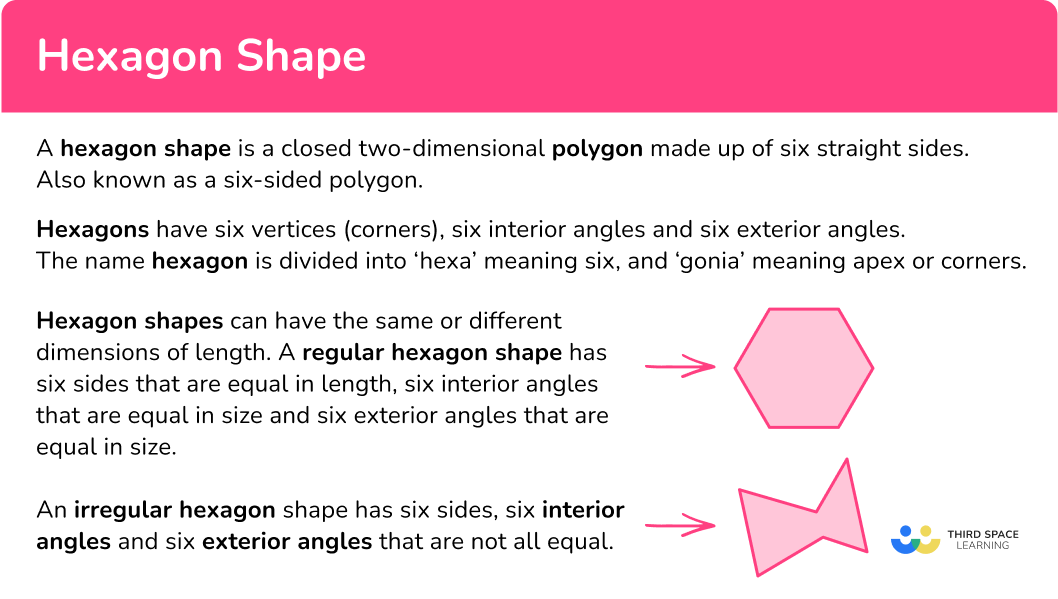## Common Core State Standards

How does this relate to Kindergarten through 4 th grade math?

• Kindergarten: Geometry (K.G.A.2)
Correctly name shapes regardless of their orientations or overall size.

Recognize and draw shapes having specified attributes, such as a given number of angles or a given number of equal faces. Identify triangles, quadrilaterals, pentagons, hexagons, and cubes.

• Grade 3: Measurement and Data (3.MD.D.8)
Solve real world and mathematical problems involving perimeters of polygons, including finding the perimeter given the side lengths, finding an unknown side length, and exhibiting rectangles with the same perimeter and different areas or with the same area and different perimeters.

Recognize a line of symmetry for a two-dimensional figure as a line across the figure such that the figure can be folded along the line into matching parts. Identify line-symmetric figures and draw lines of symmetry.

## How to identify a hexagon shape

In order to identify hexagon shapes:

1. Look for the characteristics of a hexagon shape.
2. State whether or not the shape is a hexagon.
3. If the shape is not a hexagon, explain what characteristics are different.

## Hexagon shape examples

### Example 1: hexagon shape identification

Look at the image below and determine if it is a hexagon shape or not.

1. Look for the characteristics of a hexagon shape.

A hexagon shape is a six-sided shape with six vertices (corners).

This shape has 8 straight sides and 8 vertices, or corners.

2State whether or not the shape is a hexagon.

This shape is not a hexagon. This shape is an octagon.

3If the shape is not a hexagon, explain what characteristics are different.

This shape has 2 more sides and 2 more vertices than a hexagon shape.

### Example 2: hexagon shape identification

Look at the image below and determine if it is a hexagon shape or not.

A hexagon shape is a six-sided shape with six vertices (corners).

This shape has 6 sides and 6 vertices.

This shape is an irregular hexagon.

## How to find perimeter of a hexagon shape

In order to calculate the perimeter of a hexagon shape:

1. Add all the side lengths or apply the formula.
2. Write the final answer with the correct units.

### Example 3: perimeter

Find the perimeter of the hexagon shape below.

The perimeter can be found by adding the lengths of all six sides.

7+8+4+6+3+9=37 \mathrm{~mm}

The perimeter of the hexagon shape is 37 \mathrm{~mm}.

### Example 4: perimeter

Find the perimeter of the regular hexagon below.

The perimeter can be found by adding the lengths of all six sides. This is a regular hexagon shape, so all sides are equal.

You can find the perimeter by adding up all sides or multiplying one side by six.

\begin{aligned}&4+4+4+4+4+4=24 \mathrm{~cm}\\\\ &4 \times 6=24 \mathrm{~cm}\end{aligned}

The perimeter of the hexagon shape is 24 \mathrm{~cm}.

### Example 5: find the missing side

Below is a regular hexagon shape. The perimeter of the shape is 36 \mathrm{~cm}. Find the missing side length, a.

The perimeter can be found by adding the lengths of all six sides. This is a regular hexagon, and all six sides are equal.

You also know that the perimeter is 36 \mathrm{~cm}. To find the side length, a, use the knowledge of multiplication facts or divide the perimeter by the number of equal sides.

6 \times a=36 \mathrm{~cm}

Using the multiplication facts, you know 6 \times 6=36, therefore, a=6cm.

a=36 \div 6=6 \mathrm{~cm}

The missing side of the hexagon is 6 \mathrm{~cm}.

### Example 6: find the missing side

Below is an irregular hexagon shape. The perimeter of the shape is 30 \mathrm{~cm}.
Find the missing side length.

The perimeter can be found by adding the lengths of all six sides. You also know that the perimeter is 30 \mathrm{~cm}.

First, add up the length of the sides.

2+2+7+1+9=21 \mathrm{~cm}

Then subtract the sum of the given sides from the given perimeter.

30-21=9 \mathrm{~cm}

The missing side of the hexagon is 9 \mathrm{~cm}.

### Teaching tips for the hexagon shape

• Display large, colorful visuals of hexagons on posters or using interactive whiteboards. This allows students to see the shape clearly and understand its properties.

• Instead of practice worksheets, give students problem-solving tasks that involve hexagons. For example, challenge them to construct a hexagon with specific dimensions and compare with other geometric shapes.

### Easy mistakes to make

• Assuming a hexagon is regular
Be careful to never assume a polygon is regular or irregular. If the question does not tell us, use the appropriate properties of polygons to identify regularity or irregularity.
For example, the hexagon below looks regular at a glance, however, you can see from the diagram that two interior angles are labeled, and they are not equal.
For a polygon to be regular, all six interior angles must be equal in size.

• Mixing up the interior or exterior angles
Be careful not to confuse interior and exterior angles. The Interior angles of a regular hexagon are formed inside the shape, where two sides meet and form a vertex (corner). Exterior angles are formed between a polygon and the extended line from the vertex (corner) of the polygon.

• Confusing diagonals and lines of symmetry
Be careful not to confuse diagonals with lines of symmetry. In a regular hexagon, the three long diagonals are also lines of symmetry, however, the short diagonals are not.

### Practice hexagon shape questions

1. Which of the following is an example of a hexagon shape?A hexagon shape is a six-sided shape with six vertices (corners).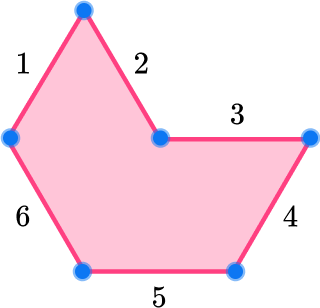This shape has 6 sides and 6 vertices. This shape is an irregular hexagon.

2. Which of the following is an example of a hexagon shape?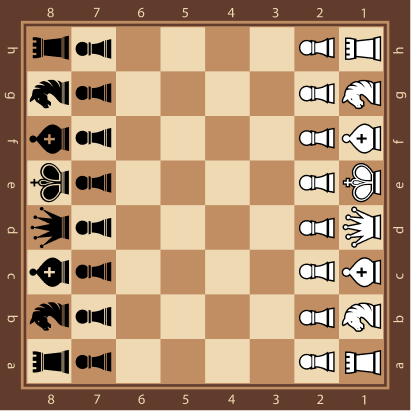A hexagon shape is a six-sided shape with six vertices (corners).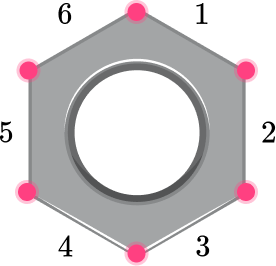This shape has 6 sides and 6 vertices. This shape is an irregular hexagon.

3. Find the perimeter of a regular hexagon shape with side length 8 \, cm.

40 \mathrm{~cm}64 \mathrm{~cm}48 \mathrm{~cm}56 \mathrm{~cm}To find the perimeter of a hexagon shape you add together the six side lengths.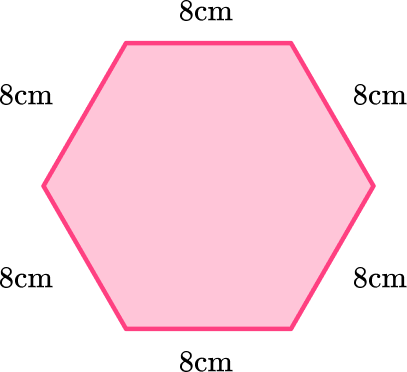This is a regular hexagon, so all six side lengths are equal, at 8 \, cm.

\begin{aligned}&8+8+8+8+8+8=48 \mathrm{~cm}\\\\ &8 \times 6=48 \mathrm{~cm}\end{aligned}

The perimeter of the hexagon is 48 \, cm.

4. Find the perimeter of the hexagon below.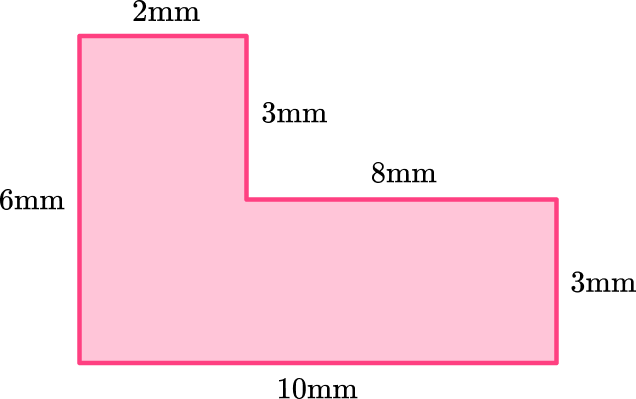30 \mathrm{~cm}29 \mathrm{~cm}15 \mathrm{~cm}32 \mathrm{~cm}To find the perimeter of a hexagon shape, you add together the six side lengths.

2+3+8+3+10+6=32 \mathrm{~cm}

The perimeter of the hexagon shape is 32 \mathrm{~cm}.

5. The perimeter of this hexagon shape is 50 \, cm. Find the missing side.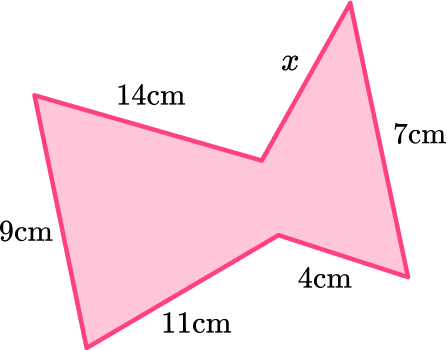14 \mathrm{~cm}5 \mathrm{~cm}3 \mathrm{~cm}11 \mathrm{~cm}To find the perimeter of a hexagon shape, you add together the six side lengths.

9+14+11+4+7=50 \mathrm{~cm}

You know five of the six sides and that the perimeter is 50 \, cm.

You can subtract the sides known from the total perimeter to find the missing side.

\text { perimeter }=50-14-9-11-4-7=5 \mathrm{~cm}

6. Below is a regular hexagon shape. The perimeter of the shape is 42 \, cm. What is the measure of the missing side length?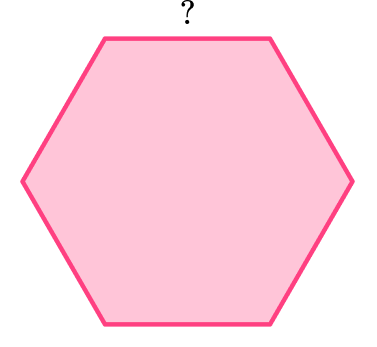6 \mathrm{~cm}5 \mathrm{~cm}7 \mathrm{~cm}8 \mathrm{~cm}To find the perimeter of a hexagon shape, you add together the six side lengths. In this case, you have the perimeter and need to find the measure of the sides.

The hexagon is a regular hexagon, so all sides are equal.

You can either divide the perimeter by 6 or use your knowledge of multiplication facts to find the missing side.

\begin{aligned}& 42 \div 6=7 \mathrm{~cm} \\\\ & 6 \times 7=42 \mathrm{~cm}\end{aligned}

The missing length of the side is 7 \mathrm{~cm}.

## Hexagon shape FAQs

How many sides does a hexagon have?

The number of sides that a hexagon has is 6. In a regular hexagon, all sides will be of equal length.

How do you find the area of a regular hexagon?

To find the area of a regular hexagon, you will use the following formula:

\text { Area }=\cfrac{\left(3 \sqrt{3} \times s^2\right) }{2}, \text { where } s=\text { length of one side of hexagon }.

The final value you obtain is the area of the regular hexagon, expressed in square units.

Is the Giant’s Causeway in Northern Ireland an example of a hexagon?

The Giant’s Causeway is an area of basalt columns that were the result of a volcanic eruption. The ancient lava cooled into hexagonal blocks or columns.

## Still stuck?

At Third Space Learning, we specialize in helping teachers and school leaders to provide personalized math support for more of their students through high-quality, online one-on-one math tutoring delivered by subject experts.

Each week, our tutors support thousands of students who are at risk of not meeting their grade-level expectations, and help accelerate their progress and boost their confidence.